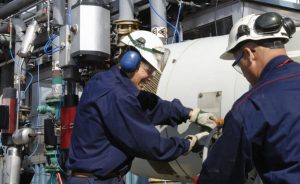# “Uncover Critical Machine Issues Before They Become Failures – Vibration Testing is the Key!”Machinery vibration analysis, validation according to ISO 10816-1 vibration velocity level chartstandard, total acceleration, crest factor, bearing damage unit, Instability, unbalance, alignment, looseness, The ISO value (in/s) is the large number at the top of the screen, which is the RMS of the vibration velocity in the frequency band 10 Hz (600 RPM) to 1 kHz (60,000 RPM), as specified by the ISO standard. Total acceleration – this is the RMS value of the total vibration in the frequency range 10 Hz to 15 kHz.This reading is shown in units of g (Earth’s gravitational constant, where g = 9.81 m/s2).
Crest Factor – this is a measure of the shape of the vibration waveform and is defined as the peak of the waveform divided by its RMS value. Crest Factor is sometimes used as a measure of the quality of a machine’s bearings. This is based on the fact that high Crest Factor is often associated with high frequency bearing noise as illustrated in the following diagrams.
Brg Noise – the final reading shown is the value of high frequency noise in Bearing Damage Units (BDU), where 100 BDU corresponds to 1g RMS vibration. This is a measure of the wear on the bearings in the equipment being monitored. The higher the number the more worn is the bearings.

Instability

Vibration in the frequency band 10 Hz (600 RPM) up to 0.75 times running speed means the vibration is occurring at less than the running speed of the machine. This not usual for a normal machine and may be an indication of an electrical fault, looseness, rubbing or some such problem that is causing uneven running. Because of the difficult of classifying them separately, these types of fault are often grouped together into the category of Instability.

Unbalance

The level of vibration in the frequency band 0.75 to 1.5 times running speed is usually indicative of how well balanced the machine is. A large vibration at the running speed indicates that the machine is out of balance. However even a very well balanced machine will usually show some vibration at the running speed but this figure should ideally be quite low (e.g. typically less than 2 mm/sec for a medium sized machine).

Alignment

Vibration in the frequency band 1.5 to 2.5 times running speed is a possible indication of misalignment. This is based on the fact that shaft misalignment can result in a double peak in the waveform due to there being two different centers of gravity (one from each shaft). In other words the accelerometer picks up a peak as each center of gravity passes by and hence there will be two positive and two negative peaks each revolution of the shaft. This will typically give rise to a vibration signal at double the running speed of the machine.

Looseness

Vibration in the frequency band 2.5 to 3.5 times running speed is a possible indication that something may be loose (e.g. loose mounting bolts, weak foundations etc) as it is not usual to see third order vibration in a machine unless there is some structural looseness that is being excited by the vibration of the machine.

Category